JEE  >  Conductance of Electrolytic Solutions & Measurement of Conductivity of Ionic Solutions

# Conductance of Electrolytic Solutions & Measurement of Conductivity of Ionic Solutions Notes | Study Chemistry for JEE - JEE

## Document Description: Conductance of Electrolytic Solutions & Measurement of Conductivity of Ionic Solutions for JEE 2022 is part of Redox Reactions & Electro Chemistry for Chemistry for JEE preparation. The notes and questions for Conductance of Electrolytic Solutions & Measurement of Conductivity of Ionic Solutions have been prepared according to the JEE exam syllabus. Information about Conductance of Electrolytic Solutions & Measurement of Conductivity of Ionic Solutions covers topics like Conductance&nbsp;, Measurement of the Conductivity of Ionic Solutions and Conductance of Electrolytic Solutions & Measurement of Conductivity of Ionic Solutions Example, for JEE 2022 Exam. Find important definitions, questions, notes, meanings, examples, exercises and tests below for Conductance of Electrolytic Solutions & Measurement of Conductivity of Ionic Solutions.

Introduction of Conductance of Electrolytic Solutions & Measurement of Conductivity of Ionic Solutions in English is available as part of our Chemistry for JEE for JEE & Conductance of Electrolytic Solutions & Measurement of Conductivity of Ionic Solutions in Hindi for Chemistry for JEE course. Download more important topics related with Redox Reactions & Electro Chemistry, notes, lectures and mock test series for JEE Exam by signing up for free. JEE: Conductance of Electrolytic Solutions & Measurement of Conductivity of Ionic Solutions Notes | Study Chemistry for JEE - JEE
 1 Crore+ students have signed up on EduRev. Have you?

Conductance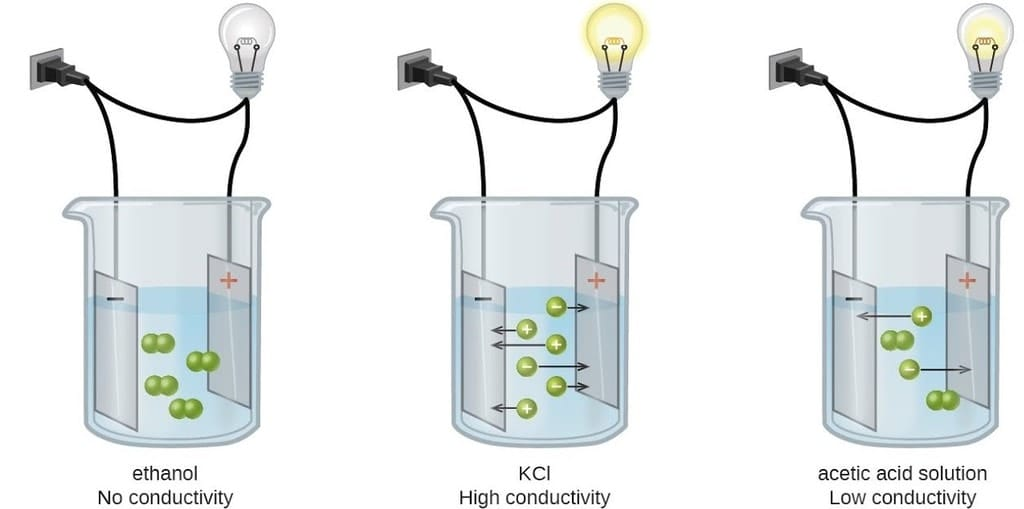Conductance/ Conductivity of Different Electrolytic Solutions

Introduction:

Both metallic and electrolytic conductors obey Ohm's law

i.e. V = IR

where V = Potential difference in volt; I Current in ampere ; R = resistance in Ohm

We know, resistance is directly proportional to length of conductor and inversely proportional to cross sectional area of the conductor.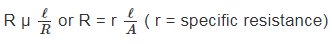Specific resistance is the resistance of a conductor having lengths of 1 cm and cross sectional area of 1 cm2.

Unit of R is ohm and unit of specific resistance is ohm cm

Reciprocal of resistance is called as conductance and reciprocal of specific resistance is called as specific conductance.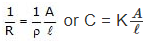where C = conductance ohm-1; K = specific conductance ohm-1 cm-1.

Ohm and siemens are other units of conductance.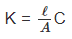Specific conductance = Cell constant x Conductance.

Specific Conductance is Conductance of 1 cm3 of an electrolyte solution.

In case of electrolytic solution, the specific conductance is defined as the conductance of a solution of definite concentration enclosed in a cell having two electrodes of unit area separated by 1 cm apart.

1. Equivalent Conductance

Equivalent conductance is the conductance of an electrolyte solution containing 1 gm equivalent of electrolyte. It is denoted by ^.

^ = K × V

( ^ = ohm-1 cm-1 × cm3 = ohm-1 cm2)

Usually concern ration of electrolyte solution is expresses as C gm equivalent per litre.

Thus, V =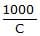{Volume having 1 gm equivalent electrolyte in the solution} Thus, ^ = K ×.

2. Molar Conductance

Molar conductance may be defined as conductance of an electrolyte solution having 1 gm mole electrolyte in a litre. It is denoted by ^m.

^m = K × V

Usually concentration of electrolyte solution is expressed as 'M' gm mole electrolyte per litre.

Thus, V =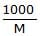Hence, ^m = K ×Relation between ^ and ^m^m = n × ^

Determination of ^m0 or ^0

A plot of ^m vs as found experimentally is as shown below graphically.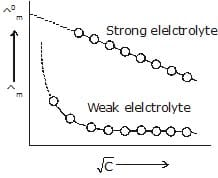the ^m vs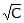plot of strong electrolyte being linear it can be extrapolated to zero concentration.

Thus, ^m values of the solution of the test electrolyte are determined at various concentrations the concentrations should be as low as good.

^m values are then plotted againstwhen a straight line is obtained. This is the extrapolated to zero concentration. The point where the straight line intersects ^m axis is ^ºm of the strong electrolyte.

However, the plot in the case weak electrolyte being non linear, shooting up suddenly at some low concentration and assuming the shape of a straight line parallel to ^m axis. Hence extrapolation in this case is not possible. Thus, ^0 of a weak electrolyte cannot be determined experimentally. It can, however, be done with the help of Kohlrausch's law to be discussed later.

Measurement of the Conductivity of Ionic Solutions

## MOLAR IONIC CONDUCTIVITY

The individual contribution of an ion, irrespective of the nature of the ion of the electrolyte in infinite dilution is called as molar conductivity.

## QUESTION ON MOLAR CONDUCTIVITY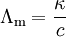The problems on molar conductivity is solved by above formula. In which k is the measured conductivity and c is the electrolyte solution .

## UNITS OF MOLAR CONDUCTIVITY

Units of molar conductivity is Siemens meter per molarity or Siemens meter per-square per mole.

## DEFINATION OF MOLAR CONDUCTIVITY

The  conductivity produced by dissolving 1 gram-mole of an electrolyte placed between two large electrodes at one centimeter apart is called as molar conductivity.
The document Conductance of Electrolytic Solutions & Measurement of Conductivity of Ionic Solutions Notes | Study Chemistry for JEE - JEE is a part of the JEE Course Chemistry for JEE.
All you need of JEE at this link: JEE

## Chemistry for JEE

213 videos|480 docs|369 tests
 Use Code STAYHOME200 and get INR 200 additional OFF

## Chemistry for JEE

213 videos|480 docs|369 tests

Track your progress, build streaks, highlight & save important lessons and more!

,

,

,

,

,

,

,

,

,

,

,

,

,

,

,

,

,

,

,

,

,

;